## Tuesday, December 31, 2013

### It's 2014 Somewhere!

In these 24 hours, a new year will begin. Here is to a happy, healthy, and successful 2014. Cheers!

Eddie

## Saturday, December 28, 2013

### HP Prime: Drawing Pixelated Pictures (DRAWICON4, DRAWICON6)

Drawing Iconic Pictures with the HP Prime

4 x 4 Pixels: DRAWICON4(number)

Entry: Real number, integer

The number is ultimately converted into a binary number, with each of the 16 bits fitting a 4 x 4 matrix. If the square is turned "on" (having a value of 1), a square will be drawn. Each space if the matrix represents a binary bit: 2^0, 2^1, 2^2, 2^3, etc. The lowest value bit is located at the bottom, right-hand corner element of the matrix.

You can specify whether the squares will be drawn in black or a specific color. The 4 x 4 matrix of bits is represented as this:

[ [ 2^15, 2^14, 2^13, 2^12 ],
[ 2^11, 2^10, 2^9, 2^8 ],
[ 2^7, 2^6, 2^5, 2^4 ],
[ 2^3, 2^2, 2^1, 2^0 ] ]

Program:

Comments followed by // are for notes and do not necessarily have to be typed.

EXPORT DRAWICON4(m)
BEGIN
// EWS 12/28/2013

// does the user want color?
LOCAL ch, R, G, B;
CHOOSE(ch,"Color?","Black","Yes");
IF ch==1 THEN
ch:=RGB(0,0,0);
ELSE
INPUT({R,G,B}, "Color Input",
{"Red","Green","Blue"},{ },{0,0,0});
ch:=RGB(R,G,B);
END;

// convert m to real to be broken down
// Type 1 is an integer (#)
IF TYPE(m)==1 THEN
m:=B→R(m);
END;

// remove negation and fraction parts (so entries like -12 and 34.55 are acceptable)
m:=ABS(IP(m));

// allow m to be text by storing it in M
LOCAL M:=m;

// check for upper limit
IF m>65535 THEN
MSGBOX("Excess of 65535!");
KILL;
END;

// break m into its binary parts and puts them in the required order
LOCAL n:=15,r,c,
mat:=MAKELIST(0,X,1,16);

REPEAT
IF 2^n≤m THEN
m:=m-2^n;
mat(n+1):=1;
END;
n:=n-1;
UNTIL n<0;
mat:=REVERSE(mat);

// list2mat(list, number of columns) - found in the catalog
mat:=list2mat(mat,4);

// drawing a border and label
RECT();
RECT_P(129,79,189,139,RGB(0,0,255),
RGB(255,255,255));
TEXTOUT_P(M,139,0,4,RGB(0,128,0));

// use the matrix to draw the squares
// pixels x = columns, y = rows
FOR r FROM 1 TO 4 DO
FOR c FROM 1 TO 4 DO

IF mat(r,c)==1 THEN
RECT_P(129+15*(c-1), 79+15*(r-1),
129+15*c, 79+15*r, ch);
END;
END;
END;

WAIT(0);

END;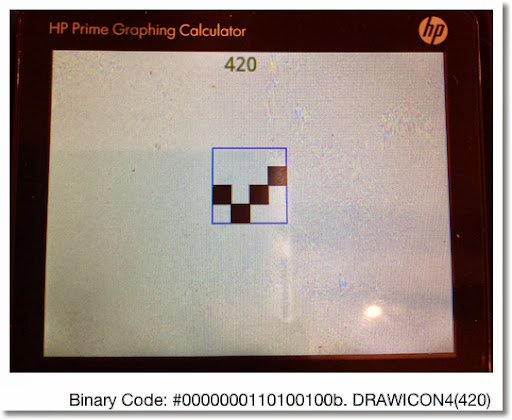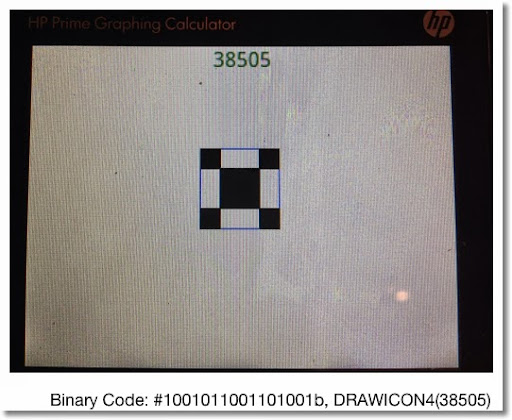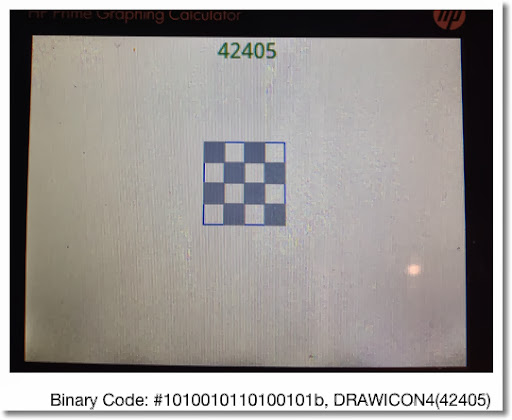Here is a 6 x 6 version:

DRAWICON6

6 x 6 Pixels: DRAWICON6(number)

Entry: Real number, integer

The program is an almost carbon-copy of DRAWICON4, except the limits have changed.

Program:

Comments followed by // are for notes and do not necessarily have to be typed.

EXPORT DRAWICON6(m)
BEGIN
// EWS 12/28/2013

// does the user want color?
LOCAL ch, R, G, B;
CHOOSE(ch,"Color?","Black","Yes");
IF ch==1 THEN
ch:=RGB(0,0,0);
ELSE
INPUT({R,G,B}, "Color Input",
{"Red","Green","Blue"},{ },{0,0,0});
ch:=RGB(R,G,B);
END;

// convert m to real to be broken down
// Type 1 is an integer (#)
IF TYPE(m)==1 THEN
m:=B→R(m);
END;

// remove negation and fraction parts (so entries like -12 and 34.55 are acceptable)
m:=ABS(IP(m));

// allow m to be text by storing it in M
LOCAL M:=m;

// check for upper limit
IF m>2^36-1 THEN
MSGBOX("Excess!");
KILL;
END;

// break m into its binary parts and puts them in the required order
LOCAL n:=35,r,c,
mat:=MAKELIST(0,X,1,36);

REPEAT
IF 2^n≤m THEN
m:=m-2^n;
mat(n+1):=1;
END;
n:=n-1;
UNTIL n<0;
mat:=REVERSE(mat);

// list2mat(list, number of columns) - found in the catalog
mat:=list2mat(mat,6);

// drawing a border and label
RECT();
RECT_P(129,79,219,169,RGB(0,0,255),
RGB(255,255,255));
TEXTOUT_P(M,139,0,4,RGB(0,128,0));

// use the matrix to draw the squares
// pixels x = columns, y = rows
FOR r FROM 1 TO 6 DO
FOR c FROM 1 TO 6 DO

IF mat(r,c)==1 THEN
RECT_P(129+15*(c-1), 79+15*(r-1),
129+15*c, 79+15*r, ch);
END;
END;
END;

WAIT(0);

END;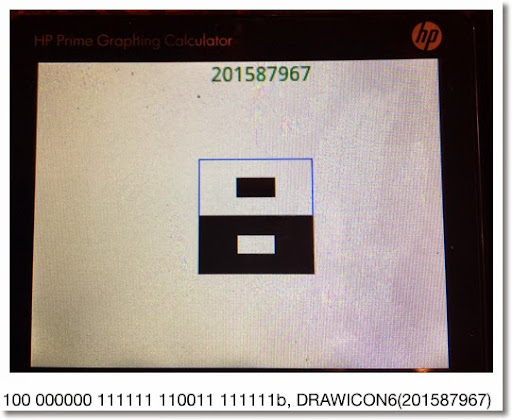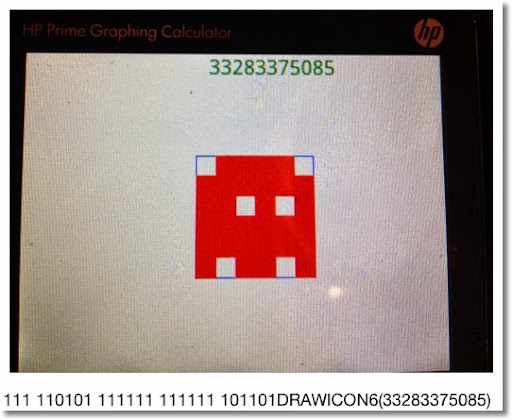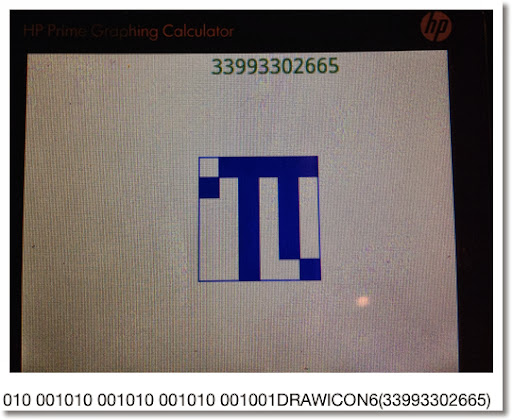Have fun and thank you as always!

I wonder how much of a challenge to do this on a TI-84?

See you next time!

Eddie

This blog is property of Edward Shore. 2013

## Tuesday, December 24, 2013

### Season Greetings!

Greetings! Thank you everybody for making this blog as successful as it is. Look for more exciting things to come into 2014. Merry Christmas and have a happy holiday season!

Eddie

This blog is property of Edward Shore. 2013

## Tuesday, December 17, 2013

### HP Prime: CAS Commands in Home Mode

(Common) CAS Commands in Home Mode
(udpated 4/17/2014)

Two things to aware of:

1. Any command from the CAS mode to be used in the Home Mode, regardless of entry, must have a "CAS." prefix. Examples include CAS.diff, CAS.simplify, etc.
2. Algebraic (symbolic) objects need single quotes surrounding them. They can be called by pressing Shift+Parenthesis Key ().
3. Not all CAS commands work as expected in Home and Programming. My focus is on the Home mode.

Simplify: CAS, 1, 1

Syntax:
Non-RPN: CAS.simplify('expression')
RPN: Not recommended - as it will numerically evaluate the expression

Expand: CAS, 1, 3

Syntax: CAS.expand('expression')
RPN: Not recommended - as it will numerically evaluate the expression

Example: CAS.expand('(X+3)^2') returns X^2+6*X+9

Factor
There are two commands for factoring:

Polynomial: CAS.Factor: CAS, 1, 4
Non-RPN Syntax: CAS.factor('expression')
Example: CAS.Factor('X^2-5*X+4') returns (X-4)*(X-1)

Integer: CAS.ifactor: CAS, 5, 2
Non-RPN Syntax: CAS.ifactor(integer)
Example: CAS.ifactor(186) returns 2*3*31

RPN Syntax:
1: integer
CAS.ifactor(1), press Enter

Differentiation: CAS, 2, 1
Non-RPN Syntax: CAS.diff('expression')

Simplification may be needed. So call up CAS.simplify, put up single quotes using Shift+Parenthesis Key, key up to the expression, press Copy (soft key), then press Enter

Example:
CAS.diff('COS(X)*SIN(X)') returns -SIN(X)*SIN(X)+COS(X)*COS(X)
CAS.simplify('-SIN(X)*SIN(X)+COS(X)*COS(X)') returns 2*COS(X)^2-1

RPN Syntax:
1: 'f(X)'
CAS.diff(1), press Enter

Update (4/17/2014):  If the above does not work, try this syntax:

CAS.diff('expression', 'variable')

Example:  CAS.diff('COS(X)*SIN(X)', 'X') returns  -SIN(X)*SIN(X)+COS(X)*COS(X)

RPN:
2:  'expression'
1:  'variable'
CAS.diff(2), press enter

Integration: CAS, 2, 2
Non-RPN Syntax: CAS.int('expression')

Simplification may be needed. So call up CAS.simplify, put up single quotes using Shift+Parenthesis Key, key up to the expression, press Copy (soft key), then press Enter

Example:
CAS.int('2*e^(4*X)') returns 2*e^(4*X)/4
CAS.simplify('2*e^(4*X)/4') returns e^(4*X)/2

Not recommended for RPN Entry

Update (4/17/2014):  If the above syntax does not work, try:

CAS.int('expression', 'variable')

Example:
CAS.int('2*X*e^(4*X)','X') returns 2*(4*X-1)/16*e^(4*X)
CAS.simplify('2*(4*X-1)/16*e^(4*X)') returns (4*X*e^(4*X) - e^(4*X))/8

Summation (Σ): CAS, 2, 5
Non-RPN Syntax: CAS.sum('expression', 'variable', start, end)
Example: CAS.sum('N^3','N',1,9) returns 2025

RPN Syntax:
4: 'expression'
3: 'variable'
2: start
1: end
CAS.sum(4) press Enter

Note: Start and End must be numerical limits.

Solving

I recommend zeros and cZeros, not solve and cSolve. The syntax is pretty much the same.

Access:
zeros: CAS, 3, 2
cZeros: CAS, 3, 4

If the equation is f(X) and we are solving for X:

Non-RPN Syntax: CAS.zeros('f(X)')

RPN Syntax:
1: 'f(X)'
CAS.zeros(1), press Enter

Example:
CAS.zeros('X^3-6') returns [1.81712059283]

If you are solving for variables other than X:

Non-RPN Syntax: CAS.zeros('expression', 'variable')

RPN Syntax:
2: 'expression'
1: 'variable'
CAS.zeros(2), press Enter

Got to include the variable!

Example:
CAS.zeros('R^3-6') returns [].
CAS.zeros('R^3-6','R') returns [1.81712059293]

Hope this helps and we will cover more of this subject in the future.

Eddie

This blog is property of Edward Shore. 2013

## Saturday, December 14, 2013

### Modular Arithmetic: Basics and Solving x MOD A = B and A MOD x = B (with A ≥ 0 and B ≥ 0)

Good to talk with you all again. Before we get back to HP Prime programming goodness, let's make a side trip into algebra land.

The Modulus Function

Definition:

X MOD Y = R where

R = remainder(X/Y) = frac(X/Y) * Y

A practical way to get the modulus is to get the remainder (in other words, what's left over) of the division X/Y.

For example:

13 MOD 5 = 3

Divide 13 by 5, we get the quotient of 2 and the remainder of 3.

Properties:

Let A and B be integers.

A MOD A = 0. Easily justified since an integer divided by itself has no remainder.

If B > A, A > 0, and B > 0, then A MOD B = A. Any integer divided by a larger integer, the remainder will be an original integer.

Example: 11 MOD 12 = 11. The division of 11/12 has the quotient of 0 and the remainder of 11.

A common way to think of the modulus function is dealing clocks and calendars (MOD 12).

x MOD A = B, A ≥ 0 and B ≥ 0

General solution: x = B + A*n, where n is any integer

Example:
x MOD 4 = 2.

Easily x = 2 is a solution since 2/4 leaves a quotient of 0 and remainder of 2.

Observe that x = 6 also works. 6/4 has a quotient of 1 and a remainder of 2.

Hence the solution is: x = 2 + 4 * n

A MOD x = B, A ≥ 0 and B ≥ 0

A way to find possible solutions is to build a table, using the good old trial and error technique. Stop when we get to A MOD A. (See the Properties section above) This can get very lengthy when A gets large. In trade, you can easily see the solutions of A MOD x = B (if solutions exist).

Example 1: 11 MOD x = 3

11 MOD 1 = 0
11 MOD 2 = 1
11 MOD 3 = 2
11 MOD 4 = 3
11 MOD 5 = 1
11 MOD 6 = 5
11 MOD 7 = 4
11 MOD 8 = 3
11 MOD 9 = 2
11 MOD 10 = 1
11 MOD 11 = 0

From this we find that the solutions are x = 4 and x = 8. A very fortunate occasion.

Example 2: 13 MOD x = 8

13 MOD 1 = 0
13 MOD 2 = 1
13 MOD 3 = 1
13 MOD 4 = 1
13 MOD 5 = 3
13 MOD 6 = 1
13 MOD 7 = 6
13 MOD 8 = 5
13 MOD 9 = 4
13 MOD 10 = 3
13 MOD 11 = 2
13 MOD 12 = 1
13 MOD 13 = 0

Darn it! No solution to 13 MOD x = 8. It happens sometimes.

Hopefully this is helpful. Thank you for your comments and questions. Honestly I can't thank you enough. Have a great day!

Eddie

This blog is property of Edward Shore. 2013

## Friday, December 6, 2013

### HP Prime Programming Tutorial #8: ARC, LINE, and Placement of Graphics

Welcome to Part 8 of the HP Prime Tutorial. Continuing in our drawing commands, we are going to feature ARC and LINE.

ARC and ARC_P: Draws a Circle or a Circular Arc

Syntax:

Cartesian:
ARC(x, y, r, angle 1*, angle 2*, color*).

Pixels:
ARC_P(x, y, r, angle 1*, angle 2*, color*)

x: x coordinate

y: y coordinate

r: length of the radius in pixels. This is why I prefer using pixel commands in my programming.

angle 1 and angle 2: If included, these two arguments draw an arc segment. The angle follows the conventional circle notation, starting with 0 (due east) going counter clockwise. Use the current angle mode.

color: color of the arc. To use it, angle 1 and angle 2 must be included.

LINE and LINE_P

Draws a line segment from (x1, y1) to (x2, y2). The color is optional.

Syntax:

Cartesian:
LINE(x1, y1, x2, y2, color*)

Pixels:
LINE_P(x1, y1, x2, y2, color*)

The next to programs CIRCLE1 and CIRCLE2 do the same thing: draw a circle, label quadrants and angels. The reason why I am posting these two is that when you are programming graphics, care must be given. Adjustments to where graphics are placed can be necessary and I hope CIRCLE1 and CIRCLE2 illustrates this.

Medium font is assumed (set in Home Settings).

The angle symbol (°) get be retrieved by pressing Shift + 9 and selecting ° from the grid.

CIRCLE1 (the "rough draft", if you will):

EXPORT CIRCLE1()
BEGIN
RECT();
TEXTOUT_P("Circle", 0, 0);
TEXTOUT_P("II",109,59);
TEXTOUT_P("90°",159,59);
TEXTOUT_P("I",209,59);
TEXTOUT_P("180°",109,109);
TEXTOUT_P("0°",209,109);
TEXTOUT_P("III",109,159);
TEXTOUT_P("270°",159,159);
TEXTOUT_P("IV",209,159);

LINE_P(0,109,318,109,RGB(16,16,16));
LINE_P(159,0,159,239,RGB(16,16,16));

ARC_P(159,109,30);
FREEZE;
END;

The results: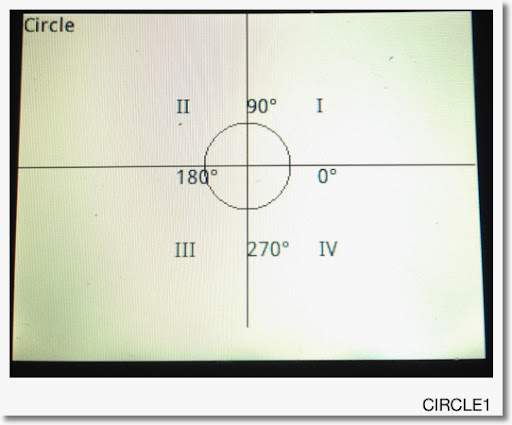This is good, but we can do better. First I am going to move the labels II, 180°, and III 10 pixels to the left, primarily so the 180° label does not touch the circle.

Second I am going to move the labels III, 270°, and IV up 10 pixels to make the bottom line look better and not "so far away" from the circle.

Although it is not necessarily, I am going to make the angle labels blue. I will need to specify the font size code at 0.

Finally, I find that the lines are too dark. I am going to make them a bit more gray.

Here is the second revision. For the purposes of this tutorial, I call this program CIRCLE2. All changes are in bold.

EXPORT CIRCLE1()
BEGIN
RECT();
TEXTOUT_P("Circle", 0, 0);
TEXTOUT_P("II",99,59);
TEXTOUT_P("90°",159,59,0,RGB(0,0,255));
TEXTOUT_P("I",209,59);
TEXTOUT_P("180°",99,109,0,RGB(0,0,255));
TEXTOUT_P("0°",209,109,0,RGB(0,0,255));
TEXTOUT_P("III",99,149);
TEXTOUT_P("270°",159,149,0,RGB(0,0,255));
TEXTOUT_P("IV",209,149);

LINE_P(0,109,318,109,RGB(128,128,128));
LINE_P(159,0,159,239,RGB(128,128,128));

ARC_P(159,109,30);
FREEZE;
END;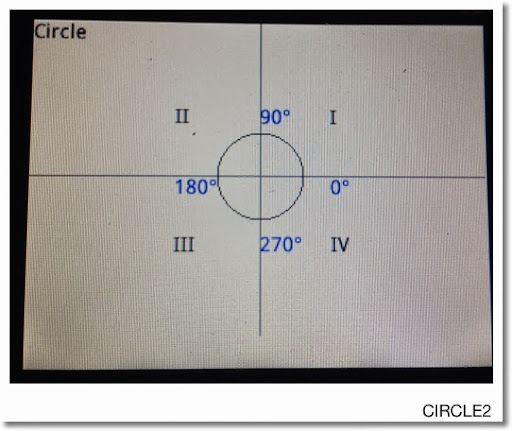A better looking picture.

This blog is property of Edward Shore. 2013

Eddie

** Note: Thanks to Dennis for pointing out my typo.  It is corrected now.  3/5/2014

### HP Prime Programming Tutorial #7: TEXTOUT

Greetings everyone. It has been too long since I last posted. I hope everyone had a fantastic Thanksgiving. For those of you who are battling cold and stormy weather, please be safe. For those of you living in the Southern Hemisphere where summer is about to begin... I'm jealous! :) I love summer.

Introduction

OK this section we will start covering some of the graphics features of the HP Prime Programming Language. We touched on graphics a bit when we used STARTAPP and STARTVIEW to call up the Plot screen of certain apps (Function, Parametric, Polar, Advanced Grpahing).

This time we are going to use drawing commands that can be used in any HP Prime app. In a sense we are creating a graphic object (GROB). The HP Prime allows for ten graphic objects, named G0 - G9. For this tutorial series, (unless specified) I am always going to use the default GROB, G0. This makes typing commands much easier.

Cartesian vs Pixel

Each graphic object operates in either one of two coordinate systems: Cartesian and pixel. If you worked with the Hewlett Packard HP 39gii calculator, this might look familiar to you.

The features of the Cartesian system (x,y) are these:

* The end points depend on the Plot variables Xmin, Xmax, Ymin, and Ymax.
* The system is familiar, having x increasing as we move to the right and y increasing as we move up. (no shocker there).
* The trade is that some (very few) drawing commands don't accommodate the Cartesian system. An example is the ARC command, which requires the radius to be in pixels.

Below is a map of the Cartesian system: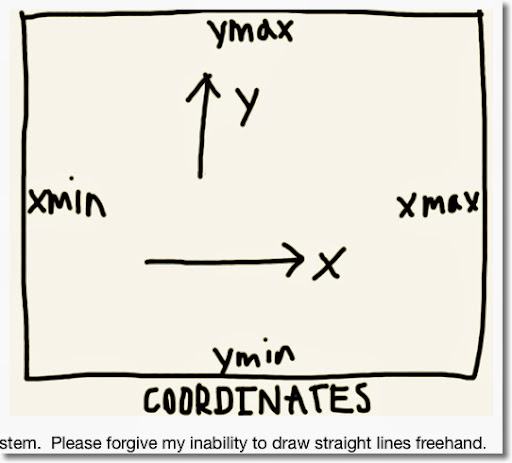The Pixel System (x,y):

* The boundaries are fixed. The pixel (0,0) is the top left hand corner, the pixel (318, 218) is the lower right hand corner.
* The value of x still increases as we go to the right. However, y increases as we go down, opposite of the Cartesian system. On the other hand, x and y are always non-negative.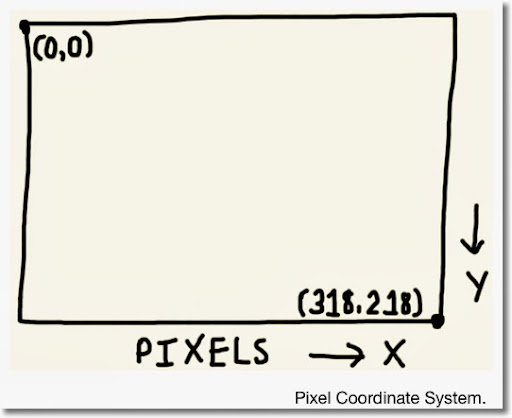The Drawing Commands

The HP Prime has two sets of drawing commands: one for the Cartesian system and one for the Pixel system. All commands for the Pixel system will have a "_P" suffix attached. For example:

LINE draws a line using Cartesian coordinates, while LINE_P draws a line using Pixel coordinates.

General Access: (in the Programming Editor)

Drawing Commands for the Pixel system: Cmds, 2. Drawing, 6. Pixels
Drawing Commands for the Cartesian system: Cmds, 2. Drawing, 7. Cartésian

Clearing the GROB screen

To clear the GROB screen, we will simply type RECT(). The wipes the screen, leaving it white. It is necessary to do this at least at the beginning of each program containing drawing commands. In a sense, RECT() is similar to PRINT().

Hint: To paint an entire screen a specific color, use RECT(color).

Showing the Graphics Screen

It is not enough to type the drawing commands. We need a command to tell the HP Prime to show the graphics. Two ways to do it are:

FREEZE: This does exactly what it says, freezes the screen. To exit, tap the screen or press ESC. Pressing Enter will re-execute the program.

Access: Cmds, 2. Drawing, 3. FREEZE

WAIT(0): This freezes the screen for an indefinite amount of time. However, pressing any button will cause the program to continue. Of course, if the last END is followed by WAIT(0), the program terminates.

Of course, you can use WAIT(n) to make the calculator wait n seconds before executing the next step.

TEXTOUT and TEXTOUT_P

TEXTOUT and TEXTOUT_P inserts text on a graphics object using Cartesian and Pixel coordinates, respectively. They are also at the bottom of the Cartesian and Pixel Drawing sub menus, respectively. (Use either the x,t,θ,n button or the up button followed by Enter).

Full Syntax (starred commands are optional):

Cartesian:
TEXTOUT(text, GROB*, x, y, font size*, text color*, width*, background color*)

Pixel:
TEXTOUT_P(text, GROB*, x, y, font size*, text color*, width*, background color*)

text: The text to be written. It can be a string, results, calculations, or any combination.

GROB*: Graphic Object G0 through G9 to be used. If left out, G0 is used.

x: x coordinate

y: y coordinate

font size*: The text font's size code. Must be used if you want text to be a color other than black. Optional. Default is the current size set by Home Settings.
0: Current font size as set by Home Settings screen.
1: Size 10 font
2: Size 12 font
3: Size 14 font
4: Size 16 font
5: Size 18 font
6: Size 20 font
7: Size 22 font

text color*: The color of the text. Use of the RGB command is advised. Optional. Default color is black.

width*: Length of the background box of the text. Optional. I usually don't use this argument.

background color*: Color of the background box. Optional. I usually don't use this argument.

Simplified Syntaxes:

Black text at default font size:
TEXTOUT(text, x, y)
TEXTOUT_P(text, x, y)

Colored text at a set font size:
TEXTOUT(text, x, y, size code, color)
TEXTOUT_P(text, x, y, size code, color)

With all this, we finally get to some programming. Since it is December, and snowing in a lot of the northern side of Earth, let's use TEXTOUT_P to draw snowflakes. I am going to use symbolize the snowflake by the asterisk, the symbol of multiplication in programming. [ × ] types *.

SNOWFLAKE

SNOWFLAKE takes one argument, which is the number of snowflakes to be drawn.

Note: Take note the order of the commands. The order regarding where to draw and generate random numbers is important to get the results you want.

Program:

EXPORT SNOWFLAKE(N)
BEGIN
LOCAL X,Y,Z,I,L0;
L0:={RGB(0,0,255),RGB(178,255,255),
RGB(30,144,255),RGB(0,255,255)};
\\ blue, light blue, dodger blue, cyan

RECT();

FOR I FROM 1 TO N DO
X:=RANDINT(0,304); \\ save some room since text takes pixels
Y:=RANDINT(0,208);
Z:=RANDINT(1,4);
Z:=L0(Z);
TEXTOUT_P("*",X,Y,2,Z);
END;

FREEZE;
END;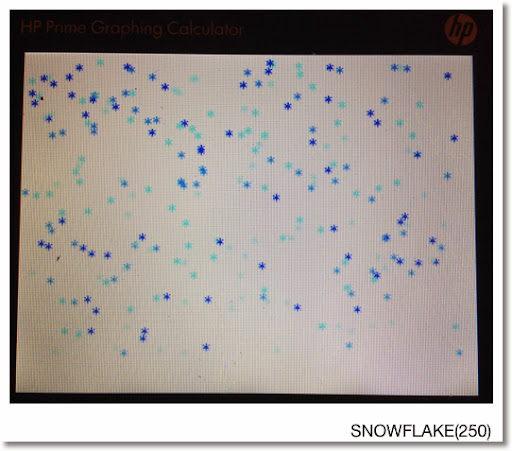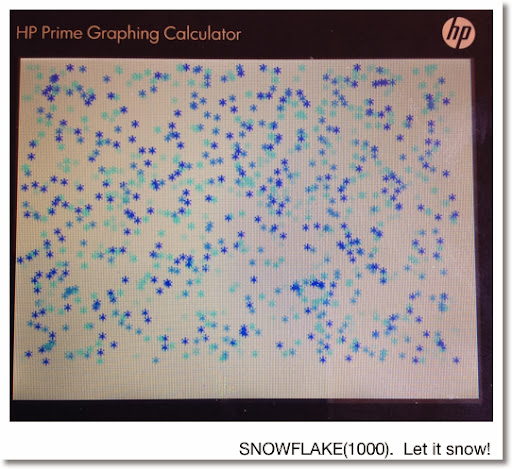Next time - we will work with ARC and LINE. Until then, Happy Day/Evening everyone!

Eddie

This blog is property of Edward Shore. 2013

And in 25 days, it will be 2014!

### TI-Nspire: Templates to Plot Functions, Parametric Equations, and Sequences

TI-Nspire:  Templates to Plot Functions, Parametric Equations, and Sequences Introduction The following are sample templates to plot functi...